# Slope

What is the slope of the line defined by the equation -2x +3y = -1 ?

k =  0.67

### Step-by-step explanation: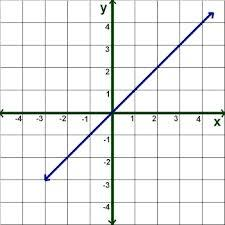Did you find an error or inaccuracy? Feel free to write us. Thank you!Tips to related online calculators
Check out our ratio calculator.
Do you have a linear equation or system of equations and looking for its solution? Or do you have a quadratic equation?

## Related math problems and questions:

• V - slopeThe slope of the line whose equation is -3x -9 = 0 is
• Slope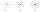Calculate the slope of a line that intersects points (-84,41) and (-76,-32).
• Slope RR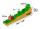A line has a rise of 2 and a run of 11. What is the slope?
• General line equationsIn all examples, write the GENERAL EQUATION OF a line that is given in some way. A) the line is given parametrically: x = - 4 + 2p, y = 2 - 3p B) the line is given by the slope form: y = 3x - 1 C) the line is given by two points: A [3; -3], B [-5; 2] D) t
• Using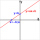Using the point-slope equation, find the equation containing (-7, 3) and slope m = -4
• Perpendicular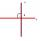Determine the slope of the line perpendicular to the line p: y = -x +4.
• SlopeWhat is the slope of a line with an inclination 6.06 rad?
• SlopeFind the slope of the line: x=t and y=1+t.
• Slope formFind the equation of a line given the point X(8, 1) and slope -2.8. Arrange your answer in the form y = ax + b, where a, b are the constants.
• Line segmentFor the line segment whose endpoints are L[-1, 13] and M[18, 2], find the x and y value for the point located 4 over 7 the distance from L to M.
• Perpendicular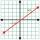What is the slope of the perpendicular bisector of line segment AB if A[9,9] and B[9,-2]?
• Golden ratio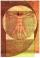Divide line of length 14 cm into two sections that the ratio of shorter to greater is same as ratio of greater section to whole length of the line.
• Line segmentCut a line segment of 15 cm into two line segments so that their lengths are in ratio 2:1. What length will each have?
• Parametric equationFind the parametric equation of a line with y-intercept (0,-4) and a slope of -2.
• AngleA straight line p given by the equation y = (-8)/(6) x +78. Calculate the size of angle in degrees between line p and y-axis.
• Find the 15Find the tangent line of the ellipse 9 x2 + 16 y2 = 144 that has the slope k = -1
• LineWrite an equation of a line parallel to To 9x + 3y = 8 That Passes Through The Point (-1, -4). Write in form ax+by=c.